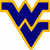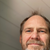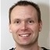+4

# With the advent of arrays, are there plans to do higher level math functions like matrix algebra?

Joe 7 years ago in IQANdesign updated by Gustav Widén (System support) 2 years ago

I want to do matrix multiplication. Any plans for this in the future?Under review

There are currently no plans to introduce matrix math, but I've added a feature request for you.

A question for you - the array channel today can only be a one-dimensional vector, do you need two-dimensional matrices?I would require two dimensional arrays for what I want to do. Thanks.+3

Wondering if Matrix math will be available. This is a feature I have a need for.Matrix math would be a vary good asset in the IQAN software enable us to do more compact equations and likely to reduce the cycle utilization. For instance, if you want to implement recursive matrix multiplication you will have to evaluate all of the matrix equations as scalar equations and then implement them separately. This is tedious to do and increases the computational time.Matrix Math functions would be very helpful. We have implemented some matrix functions using the math channels but this is tedious and requires many channels/compute time and memory, not very efficient.